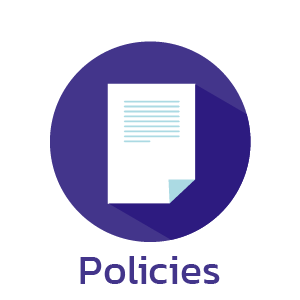﻿ Year 3 - Goodmayes Primary School

# Year 3# Curriculum Map

Outcome

• Number and place value
• Count in multiples 4 ,8, 50 and 100
• Recognise the place value of each digit in a three-digit number
• Find 10 or 100 more or less than a given number
• Order and compare numbers up to 1000
• Identify, represent and estimate numbers using different representations
• Read and write numbers up to 1000 in numerals and words
• Solve number and practical problems that involve all of the above with increasingly large positive numbers

Outcome

• Add numbers mentally: Three-digit number and ones Three-digit number and tens Three-digit number and hundreds
• Add numbers with up to three digits using formal written methods (see calculation policy)
• Subtract numbers with up to three digits using formal written methods (see calculation policy)
• Solve problems, including missing number problems using number facts, place value and more complex addition and subtraction
• Estimate and use inverse operations to check answers to a calculation

Outcome

• Multiplication and division
• Recall multiplication and division facts for tables for the 3,4, and 8 times table
• Recap multiplication and division facts for 2, 5 and 10 times table
• Write and calculate mathematical statements for multiplication and division using the multiplication tables they know
• Multiply two-digit by one-digit numbers using mental methods progressing to formal written methods (see calculation policy)
• Divide two-digit by one-digit numbers using mental methods progressing to formal written methods (see calculation policy)
• Solve problems, including missing number problems, involving multiplication and division, including integer scaling problems and harder correspondence problems such as n objects are connected to m objects

Outcome

• Fractions
• Count up or down in hundredths; recognise that tenths arise when dividing an object into 10 equal parts and in dividing one-digit numbers or quantities by 10
• Recognise, find and write fractions of a discrete set of objects: unit fractions and non-unit fraction with small denominators
• Recognise and use fractions as numbers: unit fractions and non-unit fractions with small denominators
• Recognise and show, using diagrams, equivalent fractions with small denominators
• Compare and order unit fractions and fractions with the same denominator
• Add and subtract fractions with the same denominator within one whole
• Solve problems that involve all of the above

Outcome

• Measurement
• Measure, compare, add and subtract: lengths (m/cm/mm); mass (kg/g); volume/capacity (l/ml)
• Measure the perimeter of simple 2D shapes
• Add and subtract amounts of money to give change, using both £ and p in practical contexts
• Tell and write the time from an analogue clock, including Roman numerals from I to XII
• Tell and write the time on 12 and 24 hour clocks
• Estimate and read the time to the nearest minute
• Record and compare time in terms of seconds, minutes and hours
• Use vocabulary such as: o’clock, am/pm, morning, afternoon, noon and midnight
• Know the number of seconds in a minute and the number of days in each month, year and a leap year
• Compare the duration of events e.g. calculate time taken by a particular event or task

Outcome

• Geometry
• Draw 2D shapes and make 3D shapes using modelling materials
• Recognise 3D shapes in different orientations and describe them
• Recognise angles as a property of shape or to describe a turn
• Identify right angles, recognise that two right angles make a half-turn, three make three-quarters of a turn and four make a compete turn
• Identify whether angles are greater than or less than a right angle

Outcome

• Statistics
• Interpret and present data using bar charts, pictograms and tables
• Solve one-step and two-step questions using information presented in a scaled bar chart, picograms and tables

Outcome

• Number
• Identify, represent and estimate numbers using different representations
• Read and write numbers up to 1000 in numerals and words
• Solve number and practical problems that involve all of the above with increasingly large positive numbers

Outcome

• Multiplication and division
• Solve problems, including missing number problems, involving multiplication and division, including integer scaling problems and harder correspondence problems such as n objects are connected to m objects
• Multiply two-digit by one-digit numbers using mental methods progressing to formal written methods (see calculation policy)
• Divide two-digit by one-digit numbers using mental methods progressing to formal written methods (see calculation policy)
Translate »